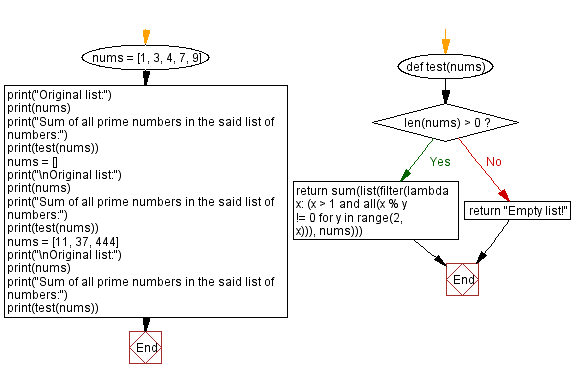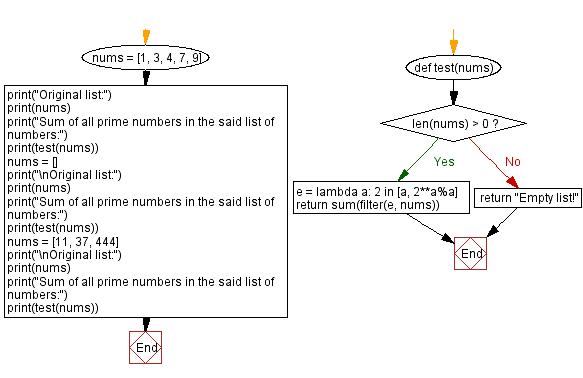﻿ Python - Sum of all prime numbers in a list of integers# Python Exercises: Sum of all prime numbers in a list of integers

## Python Math: Exercise-94 with Solution

From Wikipedia,
A prime number (or a prime) is a natural number greater than 1 that is not a product of two smaller natural numbers. A natural number greater than 1 that is not prime is called a composite number. For example, 5 is prime because the only ways of writing it as a product, 1 × 5 or 5 × 1, involve 5 itself. However, 4 is composite because it is a product (2 × 2) in which both numbers are smaller than 4.

Write a Python program to calculate the sum of all prime numbers in a given list of positive integers.

Sample Data:
([1, 3, 4, 7, 9]) -> 10
([]) -> Empty list!
([11, 37, 444]) -> 48

Sample Solution-1:

Python Code:

``````def test(nums):
if len(nums) > 0:
return sum(list(filter(lambda x: (x > 1 and all(x % y != 0 for y in range(2, x))), nums)))
return "Empty list!"

nums = [1, 3, 4, 7, 9]
print("Original list:")
print(nums)
print("Sum of all prime numbers in the said list of numbers:")
print(test(nums))
nums = []
print("\nOriginal list:")
print(nums)
print("Sum of all prime numbers in the said list of numbers:")
print(test(nums))
nums = [11, 37, 444]
print("\nOriginal list:")
print(nums)
print("Sum of all prime numbers in the said list of numbers:")
print(test(nums))
```
```

Sample Output:

```Original list:
[1, 3, 4, 7, 9]
Sum of all prime numbers in the said list of numbers:
10

Original list:
[]
Sum of all prime numbers in the said list of numbers:
Empty list!

Original list:
[11, 37, 444]
Sum of all prime numbers in the said list of numbers:
48
```

Flowchart:## Visualize Python code execution:

The following tool visualize what the computer is doing step-by-step as it executes the said program:

Sample Solution-2:

Python Code:

``````def test(nums):
if len(nums) > 0:
e = lambda a: 2 in [a, 2**a%a]
return sum(filter(e, nums))
else:
return "Empty list!"

nums = [1, 3, 4, 7, 9]
print("Original list:")
print(nums)
print("Sum of all prime numbers in the said list of numbers:")
print(test(nums))
nums = []
print("\nOriginal list:")
print(nums)
print("Sum of all prime numbers in the said list of numbers:")
print(test(nums))
nums = [11, 37, 444]
print("\nOriginal list:")
print(nums)
print("Sum of all prime numbers in the said list of numbers:")
print(test(nums))
```
```

Sample Output:

```Original list:
[1, 3, 4, 7, 9]
Sum of all prime numbers in the said list of numbers:
10

Original list:
[]
Sum of all prime numbers in the said list of numbers:
Empty list!

Original list:
[11, 37, 444]
Sum of all prime numbers in the said list of numbers:
48
```

Flowchart:## Visualize Python code execution:

The following tool visualize what the computer is doing step-by-step as it executes the said program:

Python Code Editor:

Have another way to solve this solution? Contribute your code (and comments) through Disqus.

Previous Python Exercise: Rearrange the digits of a number.
Next Python Exercise: File Exercises Home.

What is the difficulty level of this exercise?

Test your Programming skills with w3resource's quiz.

﻿

## Python: Tips of the Day

Getting sorted iterable (can sort by "compare" function):

```>>> a = [1, 2, -3]
>>> sorted(a)
[-3, 1, 2]

>>> sorted(a,key=abs)
[1, 2, -3]
```